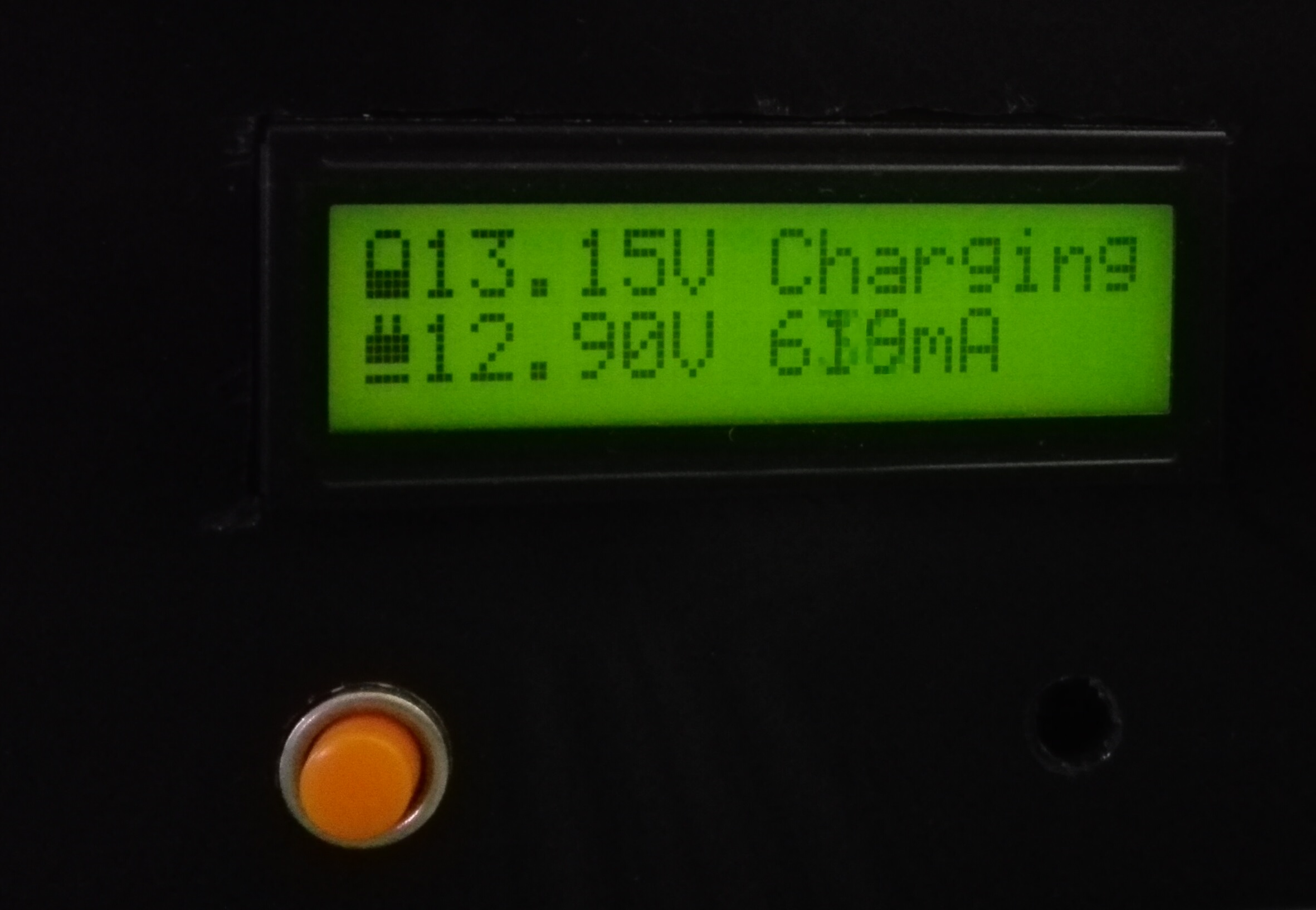# Measuring Voltage using arduinoIf you want to monitor a voltage level that is higher than the voltage that arduino input can handle, you will just need to use a voltage divider.

Basically you will only need a voltage divider if you are measuring voltage that is greater than the max input voltage of your arduino.“Vin” is where you will input the voltage to be measured. R1 and R2 forms a voltage divider to decrease voltage of the Vin. The value of R1 will be based on the max voltage that your Vin can reach.

Let R2 = 100k, you can choose higher value but check the computed value of R1 will be easy to find.

The maximum voltage that this arduino nano can handle is 5V so we will use that to compute for the value of R1. R1 is computed such that Va0 level is 5V at Maximum input voltage.

Computing for R1

For instance you are expecting the maximum input voltage is 15V, add about 10% to that to have a little headroom. So you will use 16.5V for your computation.

Vinmax = 16.5V
Va0max = 5V

R1 = (Vinmax – Va0max)/Iin

Iin = Va0max/R2

R1 = (R2(Vinmax – Va0max))/Va0max

R1 = R2((Vinmax/Va0max)-1)

replacing the value to the equation yields:

R1 = (100k)((16.5/5)-1)

R1 = 230k

Computing on software

Arduino will give you the digital value and not the actual voltage value so you need to compute and consider the voltage divider to be able to get the actual input voltage.

Actual voltage is computed as:

where:

adc_ref – ADC reference voltage. it is 5V default on arduino nano. This can be changed via program

And consider the voltage divider:

Va0 = Vin(R2/(R1+R2))

Vin = (Va0(R1+R2))/R2

Vin = (R1Va0 + R2Va0)/R2

Vin = Va0((R1/R2) + 1)

And since Va0 = Vadc then…

Now let’s put it into practice:

double Vin;
int R1;
int R2;

R1 = 230; //k
R2 = 100; //k

Displaying it into the LCD

// include the library code:
#include <LiquidCrystal.h>// initialize the library with the numbers of the interface pins – The numbers
LiquidCrystal lcd(7, 6, 5, 4, 3, 2);

double Vin;
int R1;
int R2;

void setup() {
R1 = 230; //k
R2 = 100; //k

// set up the LCD’s number of columns and rows:
lcd.begin(16, 2);
// Print a message to the LCD
lcd.print(“hello, world!”);
}

void loop() {